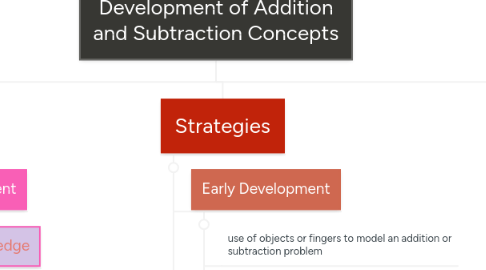# Development of Addition and Subtraction ConceptsAshley Hodkinson
Get Started. It's FreeDevelopment of Addition and Subtraction Concepts## 1. Big Ideas

### 1.1. Early Development

1.1.1. Prior Knowledge

1.1.2. understanding of part-whole development

1.1.3. two or more parts can be combined to create a whole (addition)

1.1.4. parts can be separated from a whole (subtraction)

1.1.5. basic facts of addition and subtraction

1.1.6. counting doubles (e.g., 3+3 and 6+6)

1.1.7. using concrete materials and algorithms

1.1.8. mental computations

### 1.2. Junior

1.2.1. developing meaningful computational strategies (e.g., problem-solving contexts, investigations)

1.2.2. understanding of relationship between part and whole

## 2. Strategies

### 2.1. Early Development

2.1.1. use of objects or fingers to model an addition or subtraction problem

2.1.2. transition from using direct modelling to counting strategies

2.1.3. counting on: when two sets of objects are added together, the student does not need to count all the objects in both sets, but instead begins with the number of objects in the first set and counts on from there

2.1.4. counting doubles: (e.g., 3+3 and 6+6)

2.1.5. base ten blocks and open number lines

### 2.2. Junior

2.2.1. students should have opportunities to develop and apply a variety of strategies, and to consider the appropriateness of strategies in various situations

2.2.2. students should continue to use models to develop understanding of mental and paper-and -pencil strategies

2.2.3. splitting strategy: numbers are decomposed according to place value and then each place-value part is added separately. Often used as a mental addition strategy

2.2.4. adding-on strategy: one addend is kept intact, while the other addend is decomposed into friendlier numbers

## 3. Models

### 3.1. Early Development

3.1.1. use of concrete and pictorial models (e.g., counters, base ten materials, number lines, tallies, hundreds charts)

### 3.2. Four main types

3.2.1. four main types of addition and subtraction problems: joining, separating, comporting and part-part-whole

3.2.2. joining problem involves increasing an amount by adding another amount (start amount, change amount, result amount)

3.2.3. separating problem involves decreasing an amount by removing another amount (start amount, change amount, result amount)

3.2.4. comparing problem involves the comparison of two quantities (smaller amount, larger amount, or the difference is unknown)

3.2.5. part-part-whole problem involves two parts that make the whole (when either part of the whole is unknown)

### 3.3. Junior

3.3.1. base ten materials: allow students to recognize the importance of adding ones to ones, tens to tens etc)

3.3.2. base ten materials are also used for modelling regrouping and developing an understanding of algorithms

3.3.3. open number lines: allow students to represent simple addition and subtraction operations

3.3.4. simple diagrams shows how numbers can be decomposed into parts, then the parts added to calculate partial sums, and then the partial sums added to calculate the final sum